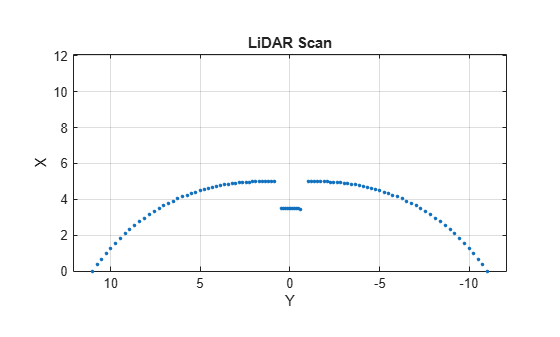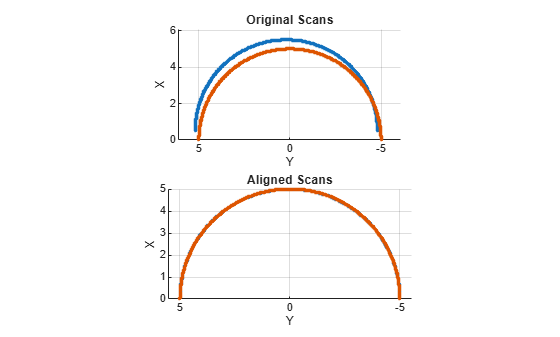# lidarScan

Create object for storing 2-D lidar scan

## Description

A `lidarScan` object contains data for a single 2-D lidar (light detection and ranging) scan. The lidar scan is a laser scan for a 2-D plane with distances (`Ranges`) measured from the sensor to obstacles in the environment at specific angles (`Angles`). Use this laser scan object as an input to other robotics algorithms such as `matchScans`, `controllerVFH`, or `monteCarloLocalization`.

## Creation

### Syntax

``scan = lidarScan(ranges,angles)``
``scan = lidarScan(cart)``

### Description

example

````scan = lidarScan(ranges,angles)` creates a `lidarScan` object from the `ranges` and `angles`, that represent the data collected from a lidar sensor. The `ranges` and `angles` inputs are vectors of the same length and are set directly to the `Ranges` and `Angles` properties.```
````scan = lidarScan(cart)` creates a `lidarScan` object using the input Cartesian coordinates as an n-by-2 matrix. The `Cartesian` property is set directly from this input.```
````scan = lidarScan(scanMsg)` creates a `lidarScan` object from a `LaserScan` (ROS Toolbox) ROS message object.```

## Properties

expand all

Range readings from lidar, specified as a vector. This vector is the same length as `Angles`, and the vector elements are measured in meters.

Data Types: `single` | `double`

Angle of range readings from lidar, specified as a vector. This vector is the same length as `Ranges`, and the vector elements are measured in radians. Angles are measured counter-clockwise around the positive z-axis.

Data Types: `single` | `double`

Cartesian coordinates of lidar readings, returned as an ```[x y]``` matrix. In the lidar coordinate frame, positive x is forward and positive y is to the left.

Data Types: `single` | `double`

Number of lidar readings, returned as a scalar. This scalar is also equal to the length of the `Ranges` and `Angles` vectors or the number of rows in `Cartesian`.

Data Types: `double`

## Object Functions

 `plot` Display laser or lidar scan readings `removeInvalidData` Remove invalid range and angle data `transformScan` Transform laser scan based on relative pose

## Examples

collapse all

Specify lidar data as vectors of ranges and angles. These values include readings outside of the sensors range.

```x = linspace(-2,2); ranges = abs((1.5).*x.^2 + 5); ranges(45:55) = 3.5; angles = linspace(-pi/2,pi/2,numel(ranges));```

Create a lidar scan by specifying the ranges and angles. Plot all points of the lidar scan.

```scan = lidarScan(ranges,angles); plot(scan)```Remove invalid points based on a specified minimum and maximum range.

```minRange = 0.1; maxRange = 7; scan2 = removeInvalidData(scan,'RangeLimits',[minRange maxRange]); hold on plot(scan2) legend('All Points','Valid Points')```Create a `lidarScan` object. Specify the ranges and angles as vectors.

```refRanges = 5*ones(1,300); refAngles = linspace(-pi/2,pi/2,300); refScan = lidarScan(refRanges,refAngles);```

Translate the laser scan by an `[x y]` offset of `(0.5,0.2)`.

`transformedScan = transformScan(refScan,[0.5 0.2 0]);`

Rotate the laser scan by 20 degrees.

`rotateScan = transformScan(refScan,[0,0,deg2rad(20)]);`

Create a reference lidar scan using `lidarScan` (Robotics System Toolbox). Specify ranges and angles as vectors.

```refRanges = 5*ones(1,300); refAngles = linspace(-pi/2,pi/2,300); refScan = lidarScan(refRanges,refAngles);```

Using the `transformScan` (Robotics System Toolbox) function, generate a second lidar scan at an `x,y` offset of `(0.5,0.2)`.

`currScan = transformScan(refScan,[0.5 0.2 0]);`

Match the reference scan and the second scan to estimate the pose difference between them.

`pose = matchScans(currScan,refScan);`

Use the `transformScan` function to align the scans by transforming the second scan into the frame of the first scan using the relative pose difference. Plot both the original scans and the aligned scans.

```currScan2 = transformScan(currScan,pose); subplot(2,1,1); hold on plot(currScan) plot(refScan) title('Original Scans') hold off subplot(2,1,2); hold on plot(currScan2) plot(refScan) title('Aligned Scans') xlim([0 5]) hold off```